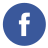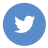### java date between two dates inclusive

java

To get the number of days between two dates in Java, including the start and end dates, you can use the until() method of the java.time.LocalDate class with the Period class.

Here’s an example:

``````import java.time.LocalDate;
import java.time.Period;

public class Main {
public static void main(String[] args) {
LocalDate start = LocalDate.of(2020, 1, 1);
LocalDate end = LocalDate.of(2020, 1, 5);

Period period = start.until(end);
int days = period.getDays();
System.out.println("Number of days: " + days);
}
}``````

The output of the example code is:

``Number of days: 4``

Note that the until() method returns a Period object, which represents a specific number of days, months, and years. In this case, we only need the number of days, so we use the getDays() method to get the number of days in the period.

You can also use the toEpochDay() method of the LocalDate class to get the number of days between two dates as a long value:

``````import java.time.LocalDate;

public class Main {
public static void main(String[] args) {
LocalDate start = LocalDate.of(2020, 1, 1);
LocalDate end = LocalDate.of(2020, 1, 5);

long days = end.toEpochDay() - start.toEpochDay();
System.out.println("Number of days: " + days);
}
}``````

The output of this code is the same as the previous example.